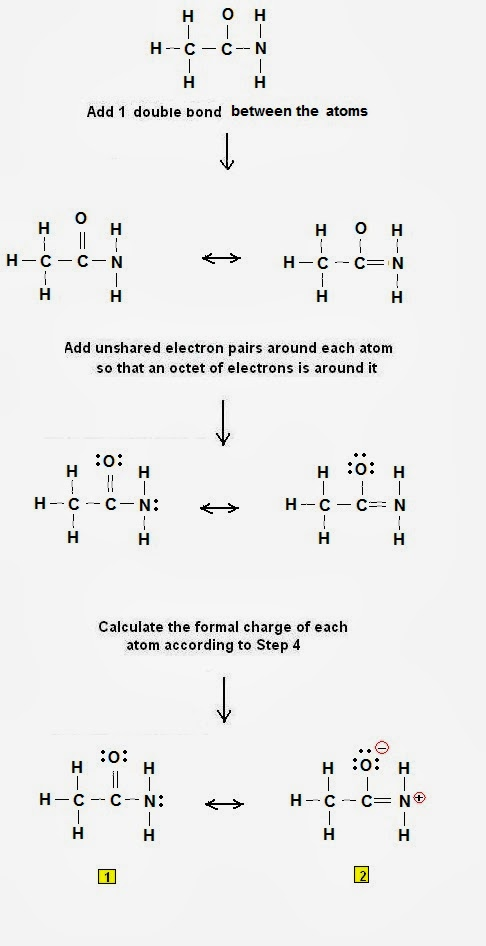Construct the electron dot structure of acetamide | Chemistry Net

# Construct the electron dot structure of acetamide

A simple procedure for writing Lewis dot structures was given in a previous post entitled “Lewis Structures and the Octet Rule”.
Several worked examples relevant to this procedure were given in previous posts please see the Sitemap - Table of Contents (Lewis Electron Dot Structures).

Let us consider the case of acetamide CH3CONH2
Acetamide has many uses and applications such as:
• As a general solvent (molten acetamide is excellent solvent for many organic and inorganic compounds)
•  in biocides
• in preparation of cosmetics and hypnotics,
• in various organic and inorganic syntheses,
• as a drug intermediate in the manufacture of ampicilline, cephaclor, cephalexin, cephradine, enalapril
• as an antacid in lacquers and cosmetics
• as a plasticiser in leather and coatings

Step 1: Connect the atoms with single bonds.Fig. 1 : Connect the atoms of acetamide with single bonds.

Step 2: Calculate the # of electrons in π bonds (pi bonds, multiple bonds)  using  formula (1)

Where n in this case is 4 since CH3CONH2  consists of nine atoms but five of them is H.

Where V = (1*3 + 4 + 4 + 6 + 5 + 1*2) = 24

Therefore, P = 6n + 2 – V = 6 * 4 + 2 – 24 = 2       So there are 2 π electrons (pi electrons) in CH3CONH2 and therefore1 double bond must be added to the structure of Step 1.

Step 3 & 4: One double bond must therefore be placed in the structure shown in Fig 1:Figure 2: Lewis structures of acetamide